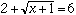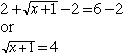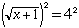Hi this is Lea. I am in secondary school. Please help with 2 plus the square root ( and under it has x plus 1) = 6 Thank you Hi Lea, What you need to do is first isolate the square root by itself on one side of the equality. Your equation is:Subtract 2 from each side to getNow square both sides:or x + 1 = 16 Finally solve for x. Whenever you solve an equation by sqaring both sides as was done in this problem it is absolutely imperative that you check your answer. Substitute the value of x into the original problem to make sure that it is a solution. Cheers, Penny Go to Math Central